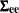# The STATESPACE Procedure

### How PROC STATESPACE Works

The design of the STATESPACE procedure closely follows the modeling strategy proposed by Akaike (1976). This strategy employs canonical correlation analysis for the automatic identification of the state space model.

Following Akaike (1976), the procedure first fits a sequence of unrestricted vector autoregressive (VAR) models and computes Akaike’s information criterion (AIC) for each model. The vector autoregressive models are estimated using the sample autocovariance matrices and the Yule-Walker equations. The order of the VAR model that produces the smallest Akaike information criterion is chosen as the order (number of lags into the past) to use in the canonical correlation analysis.

The elements of the state vector are then determined via a sequence of canonical correlation analyses of the sample autocovariance matrices through the selected order. This analysis computes the sample canonical correlations of the past with an increasing number of steps into the future. Variables that yield significant correlations are added to the state vector; those that yield insignificant correlations are excluded from further consideration. The importance of the correlation is judged on the basis of another information criterion proposed by Akaike. See the section Canonical Correlation Analysis Options for details. If you specify the state vector explicitly, these model identification steps are omitted.

After the state vector is determined, the state space model is fit to the data. The free parameters in the,, andmatrices are estimated by approximate maximum likelihood. By default, theandmatrices are unrestricted, except for identifiability requirements. Optionally, conditional least squares estimates can be computed. You can impose restrictions on elements of theandmatrices.

After the parameters are estimated, the Kalman filtering technique is used to produce forecasts from the fitted state space model. If differencing was specified, the forecasts are integrated to produce forecasts of the original input variables.